# Ion Trap Developments

Quadrupolar ion traps are common mass analyzers because of their ability to perform tandem mass spectrometry and low vacuum requirements. The rise of these instruments as mass analyzers was set off by the implementation of mass selective instability. This is done by raising the radio frequency (rf) trapping voltage so that ions are ejected in increasing m/z order.  The motion of ions in a quadrupole is dictated by the Mathieu equation. The trapping parameters, a and q, are commonly used to define the stability of ions within the trap. These trapping parameters’ equations are shown below: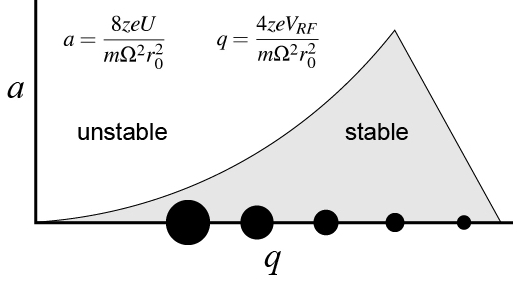Figure 1. Mathieu parameters

where z is the number of charges, e is the elementary charge, U is the DC potential on the rods, Vrf is the zero-to-peak rf potential, Ω, is the angular frequency (2πf is the rf frequency), rois the half distance between rods, m is the mass of the ion in kilograms. Quadrupolar ion traps typically operate with no DC potential applied to the rods (a = 0), which means all ions can be represented on only the q-axis. It can be seen from these equations how a linear in time scan of the trapping voltage would provide a linear in time scan of m/z.  Ions trapped in an quadrupolar field will oscillate with a specific frequency.  This fundamental frequency is related to another parameter, β.  β is approximated by specific a and q values. It was shown that an ion will resonant with an applied ac matching their fundamental frequency increases the amplitude of the ion’s trajectory until it is unstable and ejected.  This was coupled to the rf ramp so that ions will successively be raised to a specific q value and resonant with the ejection frequency.  Our lab has implemented a frequency sweep at a constant trapping voltage to allow ions to be ejected at their secular frequency. The sweep is created in a manner so that the m/z excited is linearly correlated to time. This allows for much easier time to m/z calibration. A comparison of the RF voltage scan vs frequency sweep is shown below: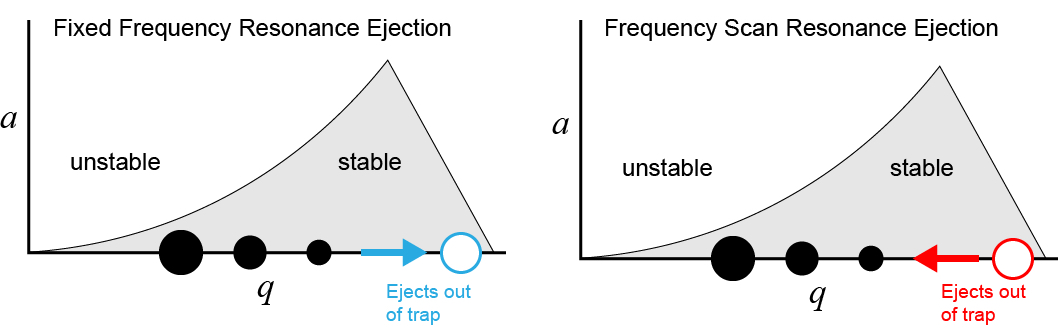Figure 2. Resonance ejection from an ion trap can be done by scanning ions to single frequency via an RF voltage ramp or scanning of the ejection frequency

Although the fixed frequency resonance ejection has been widely implemented, the frequency scan version has not seen much attention. The advantage of using the frequency scanned version is that ion’s will have the same fundamental frequency throughout the scan. This allows for mass-selective activation throughout the scan. This is more crucial in tandem mass spectrometry experiments in which a precursor ion is fragmented to produce a product ion. There are four common tandem mass spectrometry experiments. They are permutations of scanning and fixing either the precursor or product ion m/z. They are outlined below: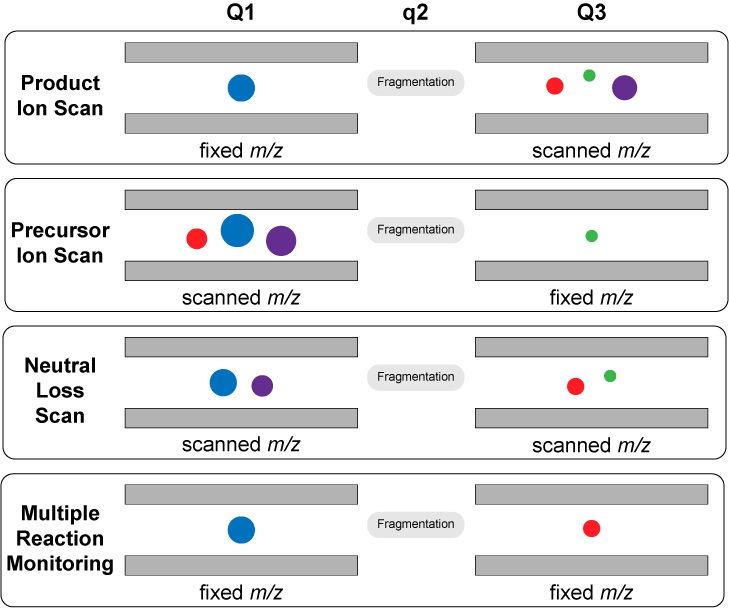Figure 3. Scan modes in tandem mass spectrometry

In the case of the fixed frequency resonance ejection, only product ion scans have been implemented. This is because all the precursor ions besides the single m/z of interest needs to be ejected so that the signal at the detector represents product ions instead of a combination of both unreacted precursor ions and product ions. A scanned frequency resonance experiment allows only the ions of interest to be activated/ejected as they will be individually activated by a waveform containing their fundamental frequency. The tandem mass spectrometry scan modes described above are implemented on a scanned frequency resonance instrument by applying two waveforms in orthogonal dimensions. The waveform that activates precursor ions is applied to the set of electrodes not in plane with the detector. This allows the precursor ions to gain kinetic energy and collide with neutral bath gas to build internal energy until the precursor ion fragments into a product ion and a neutral. A second waveform is applied to the electrodes in plane with the detector, so that any product ions activated by the waveform will be ejected into the detector. The waveform can be composed of a fixed individual frequency or the previously mentioned frequency sweep so that ions of increasing m/z will be excited linearly with time. Recent developments have allowed the waveforms to activate multiple ions in the same waveform. This allows for simultaneous detection of two different product ions.  This allows for two scan modes to be performed at the same time. The issue with this experiment is that the two different product ions being detected cannot be differentiated between at the detector.  This challenge was overcame by encoding beat frequencies to the ejection waveform so that ejection events were modulated by specific beats.  Fourier analysis of the individual peaks allows for the differentiation of simultaneous scan modes. A visual depiction of the experiment is shown below: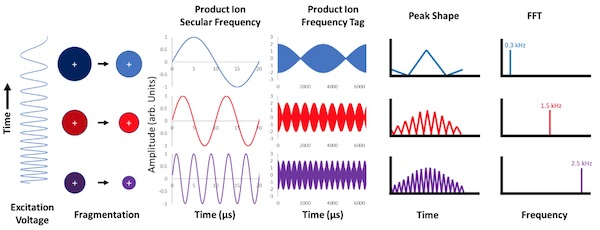Figure 4. Schematic of beat frequency encoding methodology

The ability to differentiate simultaneous scan modes is shown below: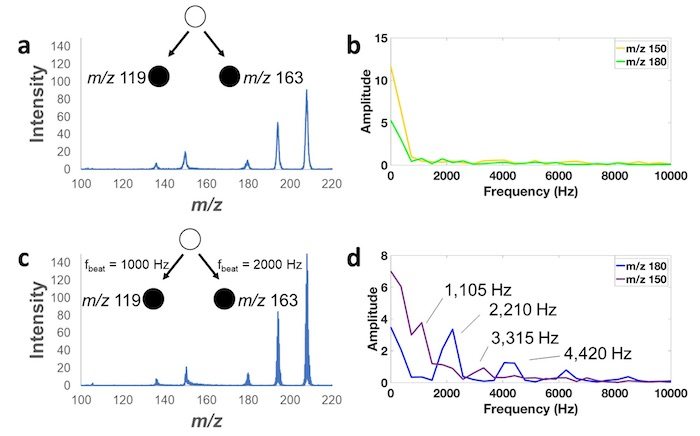Figure 5. (a) A double precursor scan of both m/z 119 and 163 (b) resulting FFTs of m/z 150 and m/z 180, (c) a double precursor scan with two different frequency tags applied to both ejection waveforms, (d) resulting FFTs of m/z 150 and 180.

#### Publications

1. Snyder, D. T.; Szalwinski, L. J.; Cooks, R. G., Mass Spectrometry | Ion Traps. In Reference Module in Chemistry, Molecular Sciences and Chemical Engineering, Elsevier: 2018.

2. Snyder, D. T.; Pulliam, C. J.; Cooks, R. G., Linear mass scans in quadrupole ion traps using the inverse Mathieu q scan. Rapid Commun. Mass Spectrom. 2016, 30 (22), 2369-2378.

3. Snyder, D. T.; Kaplan, D. A.; Danell, R. M.; van Amerom, F. H. W.; Pinnick, V. T.; Brinckerhoff, W. B.; Mahaffy, P. R.; Cooks, R. G., Unique capabilities of AC frequency scanning and its implementation on a Mars Organic Molecule Analyzer linear ion trap. Analyst 2017, 142 (12), 2109-2117.

4. Snyder, D. T.; Szalwinski, L. J.; Cooks, R. G., Simultaneous and Sequential MS/MS Scan Combinations and Permutations in a Linear Quadrupole Ion Trap. Analytical Chemistry 2017, 89 (20), 11053-11060.

5. Snyder, D. T.; Szalwinski, L. J.; Wells, J. M.; Cooks, R. G., Logical MS/MS scans: a new set of operations for tandem mass spectrometry. Analyst 2018, 143 (22), 5438-5452.